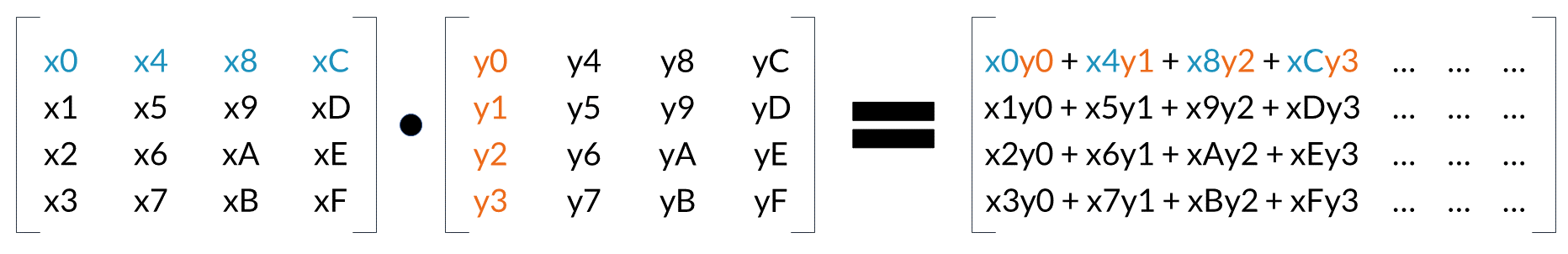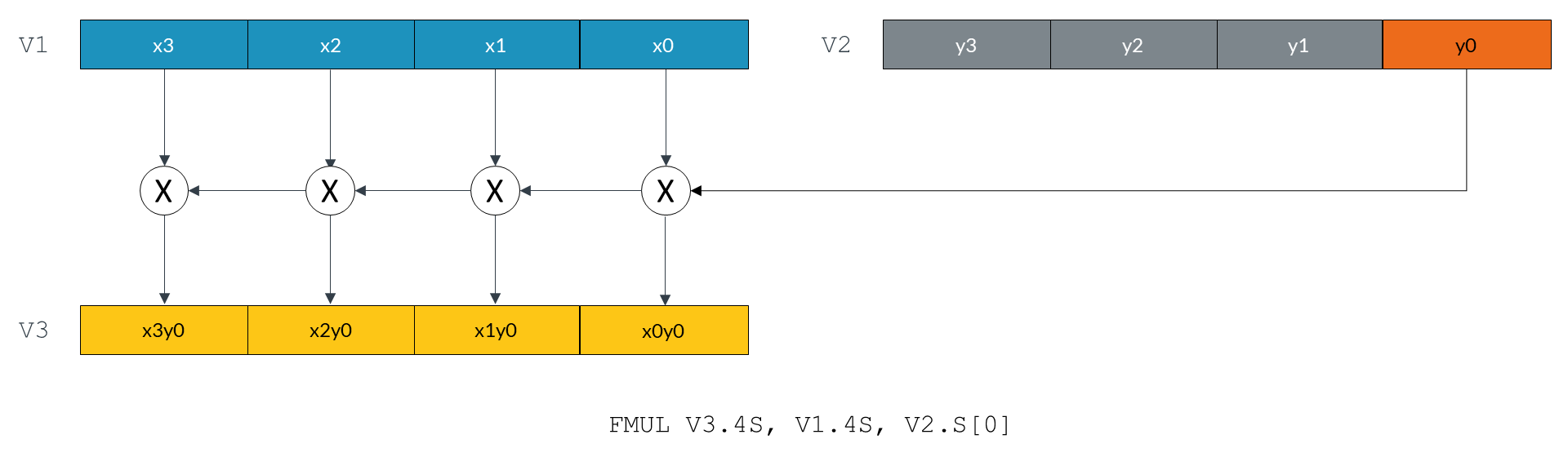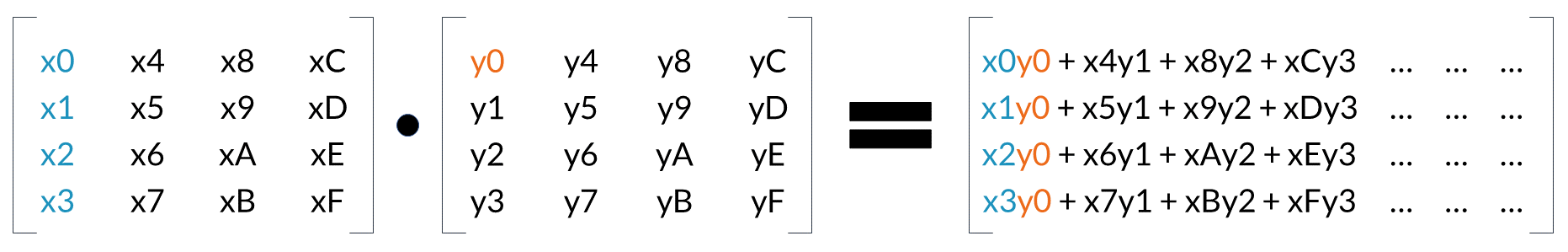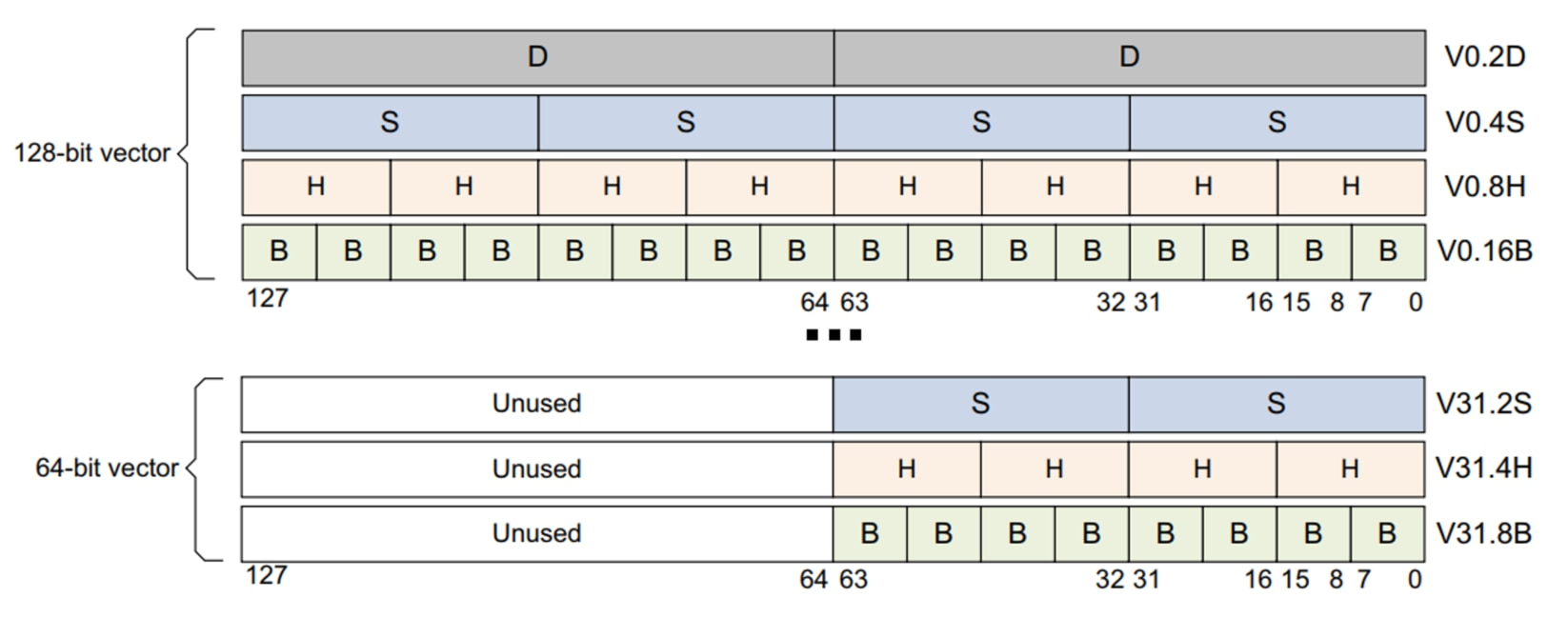## Matrix multiplication

In this section of the guide, we look at how you can use Neon to perform an example data processing task. Specifically, we show you how to efficiently multiply four-by-four matrices together, an operation frequently used in the world of 3D graphics. We assume that the matrices are stored in column-major order because OpenGL ES uses this format.

Note: Download the code for the functions that are described in this section here: matrix_asm_a64.s.zip

### The algorithm

First, we will look at the algorithm that multiplies two matrices together. We expand the calculation to examine the matrix multiplication operation in detail, then identify operations that we can implement using Neon instructions.

The following diagram shows how to calculate the first column of results when multiplying two matrices together:Look at the first element in the result matrix. Every element in the first row of the first matrix (blue) is multiplied by the corresponding element in the first column of the second matrix (orange). We accumulate the results to give the first result value. This process is repeated for all the remaining elements in the result matrix.

The following diagram shows how we can use the Neon FMUL vector-by-scalar multiplication instruction to calculate these results:The `FMUL` instruction in the preceding diagram multiplies every element of the vector in the V1 register by the scalar value in lane 0 of the V2 register. The instruction then stores the resulting vector in the V3 register.

The following diagram shows how this single instruction calculates the first term for each of the values in the first column of the result matrix:We can use the same method to calculate the remaining terms. However, this time we will use the `FMLA` multiply and accumulate instruction to sum the terms.

Because we are operating on the columns of the first matrix and producing a column of results, reading and writing elements is a linear operation. Interleaving load or store instructions are not required.

### Neon registers and data size

The Neon register file is a collection of registers that can be accessed as either 64-bit or 128-bit registers.

The number of lanes in a Neon vector depends on the size of the vector and the data elements in the vector. The following diagram shows the different ways that you can arrange and access data in Neon registers:This guide examines two different implementations of the matrix multiplication algorithm. Each implementation performs multiplication in a different way:

• The floating-point implementation operates on values using the 32-bit floating-point format.

Multiplying two 32-bit floating-point numbers gives a result that is another 32-bit number. This means that the floating-point implementation uses the 4S vector lane format throughout.

• The fixed-point implementation operates on values using the 16-bit Q1.14 fixed-point format.

Multiplying two 16-bit Q1.14 fixed-point format numbers together gives a 32-bit result that must be narrowed to 16 bits. This means that we can use the 4H vector lane format for the 16-bit input and result values, but the 4S vector lane format for the intermediate multiplication result.

### Floating-point implementation

The floating-point implementation multiplies two matrices that contain 32-bit floating-point numbers.

The implementation has three stages:

1. Load the matrix data from memory to Neon registers.
2. Perform the matrix multiplication operation.
3. Store the result matrix back to memory.

The following code shows how we load the data into the Neon registers:

```LD1  {V0.4S, V1.4S, V2.4S, V3.4S}, [X1]
LD1  {V4.4S, V5.4S, V6.4S, V7.4S}, [X2] ```

Our matrices are stored in column-major order. This means that the column data is stored linearly in memory. We use the `LD1` instruction to load data from memory into the Neon registers V0 - V7.

Neon provides 32 registers. Each register is 128 bits wide. We can load all the elements from both input matrices into registers, and still have registers left over to use as accumulators. In this implementation, registers V0-V3 hold the 16 elements from the first matrix, and registers V4-V7 hold the 16 elements from the second matrix. Each 128-bit register holds four 32-bit values, representing an entire matrix column.

Similarly, the following code shows how we use the `ST1` instruction to store the result back to memory:

`ST1  {V8.4S, V9.4S, V10.4S, V11.4S}, [X0]`

The following code shows how we calculate a column of results using just four Neon multiply instructions:

```FMUL V8.4S, V0.4S, V4.S           // rslt col0  = (mat0 col0) * (mat1 col0 elt0)
FMLA V8.4S, V1.4S, V4.S           // rslt col0 += (mat0 col1) * (mat1 col0 elt1)
FMLA V8.4S, V2.4S, V4.S           // rslt col0 += (mat0 col2) * (mat1 col0 elt2)
FMLA V8.4S, V3.4S, V4.S           // rslt col0 += (mat0 col3) * (mat1 col0 elt3)```

The first `FMUL` instruction implements the operation that is highlighted in the previous diagram. Matrix elements x0, x1, x2, and x3 (in the four lanes of register V0) are each multiplied by y0 (element 0 in register V4), and the result stored in V8.

Subsequent `FMLA` instructions operate on the other columns of the first matrix, multiplying by corresponding elements of the first column of the second matrix. Results are accumulated into V8 to give the first column of values for the result matrix.

If we only need to calculate a matrix-by-vector multiplication, the operation is now complete. However, to complete the matrix-by-matrix multiplication, we must execute three more iterations. These iterations use values y4 to yF in registers V5 toV7.

The following code shows the full implementation of a four-by-four floating-point matrix multiply:

```matrix_mul_float:
LD1  {V0.4S, V1.4S, V2.4S, V3.4S}, [X1] // load all 16 elements of matrix 0 into
//  V0-V3, four elements per register
LD1  {V4.4S, V5.4S, V6.4S, V7.4S}, [X2] // load all 16 elements of matrix 1 into
//  V4-V7, four elements per register

FMUL V8.4S, V0.4S, V4.S     // rslt col0  = (mat0 col0) * (mat1 col0 elt0)
FMUL V9.4S, V0.4S, V5.S     // rslt col1  = (mat0 col0) * (mat1 col1 elt0)
FMUL V10.4S, V0.4S, V6.S    // rslt col2  = (mat0 col0) * (mat1 col2 elt0)
FMUL V11.4S, V0.4S, V7.S    // rslt col3  = (mat0 col0) * (mat1 col3 elt0)

FMLA V8.4S, V1.4S, V4.S     // rslt col0 += (mat0 col1) * (mat1 col0 elt1)
FMLA V9.4S, V1.4S, V5.S     // rslt col1 += (mat0 col1) * (mat1 col1 elt1)
FMLA V10.4S, V1.4S, V6.S    // rslt col2 += (mat0 col1) * (mat1 col2 elt1)
FMLA V11.4S, V1.4S, V7.S    // rslt col3 += (mat0 col1) * (mat1 col3 elt1)

FMLA V8.4S, V2.4S,  V4.S    // rslt col0 += (mat0 col2) * (mat1 col0 elt2)
FMLA V9.4S, V2.4S,  V5.S    // rslt col1 += (mat0 col2) * (mat1 col1 elt2)
FMLA V10.4S, V2.4S, V6.S    // rslt col2 += (mat0 col2) * (mat1 col2 elt2)
FMLA V11.4S, V2.4S, V7.S    // rslt col3 += (mat0 col2) * (mat1 col2 elt2)

FMLA V8.4S, V3.4S, V4.S     // rslt col0 += (mat0 col3) * (mat1 col0 elt3)
FMLA V9.4S, V3.4S, V5.S     // rslt col1 += (mat0 col3) * (mat1 col1 elt3)
FMLA V10.4S, V3.4S, V6.S    // rslt col2 += (mat0 col3) * (mat1 col2 elt3)
FMLA V11.4S, V3.4S, V7.S    // rslt col3 += (mat0 col3) * (mat1 col3 elt3)

ST1  {V8.4S, V9.4S, V10.4S, V11.4S}, [X0] // store all 16 elements of result

### Fixed-point implementation

Using fixed-point arithmetic for calculations is often faster than using floating-point arithmetic. Fixed-point arithmetic requires less memory bandwidth than floating-point arithmetic to read and write values that use fewer bits. Because fixed-point arithmetic uses integer data types, multiplication of fixed-point values is usually quicker than the same operations applied to floating point numbers.

However, when using fixed-point arithmetic, you must choose the representation carefully, so that you can avoid overflow or saturation. At the same time, you must preserve the degree of precision in the results that your application requires.

Implementing a matrix multiply using fixed-point values is very similar to floating-point. This example uses Q1.14 fixed-point format, but the operations are similar for other formats. Adapting this example to another fixed-point format might only require a change to the final shift that is applied to the accumulator.

Our fixed-point implementation uses a macro to perform the matrix multiplication, as shown in the following code:

```  .macro mul_col_s16 res_d, col_d
SMULL V12.4S, V0.4H, \col_d\().H // multiply col element 0 by matrix col 0
SMLAL V12.4S, V1.4H, \col_d\().H // multiply col element 0 by matrix col 1
SMLAL V12.4S, V2.4H, \col_d\().H // multiply col element 0 by matrix col 2
SMLAL V12.4S, V3.4H, \col_d\().H // multiply col element 0 by matrix col 3
SQSHRN \res_d\().4H, V12.4S, #14    // shift right and narrow accumulator into
//  Q1.14 fixed-point format, with saturation
.endm```

Comparing the fixed-point implementation to the floating-point implementation, the major differences are:

• Matrix values are now 16-bit instead of 32-bit. Because of this difference, we use the 4H configuration to store four 16-bit values in the lower 64 bits of the 128-bit Neon register.
• The result of multiplying two 16-bit numbers is a 32-bit number. We use the signed multiply long `SMULL` and signed multiply-add long `SMLAL` instructions to store the results in the 32-bit 4S lane configuration of the Neon register.
• The final result matrix must contain 16-bit values, but the accumulators contain 32-bit values. We obtain a 16-bit result using the `SQSHRN` signed saturating shift right narrow instruction. This instruction adds the correct rounding value to each element, shifts it right, and saturates the result to the new, narrower element size.

The following code shows the full implementation of a four-by-four fixed-point matrix multiply:

```  .macro mul_col_s16 res_d, col_d
SMULL V12.4S, V0.4H, \col_d\().H   // multiply col element 0 by matrix col 0
SMLAL V12.4S, V1.4H, \col_d\().H   // multiply col element 0 by matrix col 1
SMLAL V12.4S, V2.4H, \col_d\().H   // multiply col element 0 by matrix col 2
SMLAL V12.4S, V3.4H, \col_d\().H   // multiply col element 0 by matrix col 3
SQSHRN \res_d\().4H, V12.4S, #14      // shift right and narrow accumulator into
//  Q1.14 fixed-point format, with saturation
.endm

.global matrix_mul_fixed
matrix_mul_fixed:
LD1  {V0.4H, V1.4H, V2.4H, V3.4H}, [X1] // load all 16 elements of matrix 0
// into V0-V3, four elements per register
LD1  {V4.4H, V5.4H, V6.4H, V7.4H}, [X2] // load all 16 elements of matrix 1
// into V4-V7, four elements per register

mul_col_s16 v8, v4                        // matrix 0 * matrix 1 col 0
mul_col_s16 v9, v5                        // matrix 0 * matrix 1 col 1
mul_col_s16 v10, v6                       // matrix 0 * matrix 1 col 2
mul_col_s16 v11, v7                       // matrix 0 * matrix 1 col 3

ST1  {V8.4H, V9.4H, V10.4H, V11.4H}, [X0] // store all 16 elements of result

### Optimized instruction scheduling

The fixed-point implementation uses a macro to perform the main multiplication operation on each matrix column. In the macro, adjacent multiply instructions write to the same register: V12. This means that each Neon pipeline must wait for each multiply to complete before it can start the next instruction. The following code repeats the macro from the fixed-point implementation:

```.macro mul_col_s16 res_d, col_d
SMULL V12.4S, V0.4H, \col_d\().H // multiply col element 0 by matrix col 0
SMLAL V12.4S, V1.4H, \col_d\().H // multiply col element 0 by matrix col 1
SMLAL V12.4S, V2.4H, \col_d\().H // multiply col element 0 by matrix col 2
SMLAL V12.4S, V3.4H, \col_d\().H // multiply col element 0 by matrix col 3
SQSHRN \res_d\().4H, V12.4S, #14    // shift right and narrow accumulator into
//  Q1.14 fixed-point format, with saturation
.endm```

If we take the instructions out of the macro and rearrange them, we can separate instructions that write to the same register. This reduces the risk of register contention and allows instructions to make efficient use of the Neon pipeline.

The following code shows how to rearrange and optimize accesses to the accumulator registers:

```SMULL V12.4S, V0.4H, V4.H
SMULL V13.4S, V0.4H, V5.H
SMULL V14.4S, V0.4H, V6.H
SMULL V15.4S, V0.4H, V7.H
SMLAL V12.4S, V1.4H, V4.H
SMLAL V13.4S, V1.4H, V5.H
SMLAL V14.4S, V1.4H, V6.H
SMLAL V15.4S, V1.4H, V7.H

SMLAL V12.4S, V2.4H, V4.H
SMLAL V13.4S, V2.4H, V5.H
SMLAL V14.4S, V2.4H, V6.H
SMLAL V15.4S, V2.4H, V7.H

SMLAL V12.4S, V3.4H, V4.H
SMLAL V13.4S, V3.4H, V5.H
SMLAL V14.4S, V3.4H, V6.H
SMLAL V15.4S, V3.4H, V7.H

SQSHRN V8.4H, V12.4S, #14
SQSHRN V9.4H, V13.4S, #14
SQSHRN V10.4H, V14.4S, #14
SQSHRN V11.4H, V15.4S, #14   ```